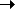Package net.flashpunk.tweens.motion Class public class QuadMotion Inheritance QuadMotionMotionTweenObject

Determines motion along a quadratic curve.

Public Properties
PropertyDefined Byactive : Boolean
If the tween should update.
Tweencomplete : Function
Tween completion callback.
Tweendelay : Number = 0
Length of time to wait before starting this tween.
Tween
distance : Number
[read-only] The distance of the entire curve.object : Object
Target object for the tween.
Motionpercent : Number
The completion percentage of the Tween.
Tweenscale : Number
[read-only] The current time scale of the Tween (after easer has been applied).
Tweenx : Number
Current x position of the Tween.
Motiony : Number
Current y position of the Tween.
Motion
Protected Properties
PropertyDefined By_object : Object
Motion_x : Number = 0
Motion_y : Number = 0
Motion
Public Methods
MethodDefined By

QuadMotion(complete:Function = null, type:uint = 0)
Constructor.cancel():void
Immediately stops the Tween and removes it from its Tweener without calling the complete callback.
Tween

setMotion(fromX:Number, fromY:Number, controlX:Number, controlY:Number, toX:Number, toY:Number, duration:Number, ease:Function = null):void
Starts moving along the curve.

setMotionSpeed(fromX:Number, fromY:Number, controlX:Number, controlY:Number, toX:Number, toY:Number, speed:Number, ease:Function = null):void
Starts moving along the curve at the speed.start():void
Starts the Tween, or restarts it if it's currently running.
Tweenupdate():void
Updates the Tween, called by World.
Tween
Public Constants
ConstantDefined ByLOOPING : uint = 1
[static] Looping Tween type, will restart immediately when it finishes.
TweenONESHOT : uint = 2
[static] Oneshot Tween type, will stop and remove itself from its core container when it finishes.
TweenPERSIST : uint = 0
[static] Persistent Tween type, will stop when it finishes.
Tween
Property Detail
 distance property
`distance:Number`  [read-only]

The distance of the entire curve.

Implementation
`    public function get distance():Number`
Constructor Detail
`public function QuadMotion(complete:Function = null, type:uint = 0)`

Constructor.

Parameters
 `complete:Function` (default = `null`) — Optional completion callback. `type:uint` (default = `0`) — Tween type.
Method Detail
 setMotion () method
` public function setMotion(fromX:Number, fromY:Number, controlX:Number, controlY:Number, toX:Number, toY:Number, duration:Number, ease:Function = null):void`

Starts moving along the curve.

Parameters

 `fromX:Number` — X start. `fromY:Number` — Y start. `controlX:Number` — X control, used to determine the curve. `controlY:Number` — Y control, used to determine the curve. `toX:Number` — X finish. `toY:Number` — Y finish. `duration:Number` — Duration of the movement. `ease:Function` (default = `null`) — Optional easer function.

 setMotionSpeed () method
` public function setMotionSpeed(fromX:Number, fromY:Number, controlX:Number, controlY:Number, toX:Number, toY:Number, speed:Number, ease:Function = null):void`

Starts moving along the curve at the speed.

Parameters

 `fromX:Number` — X start. `fromY:Number` — Y start. `controlX:Number` — X control, used to determine the curve. `controlY:Number` — Y control, used to determine the curve. `toX:Number` — X finish. `toY:Number` — Y finish. `speed:Number` — Speed of the movement. `ease:Function` (default = `null`) — Optional easer function.